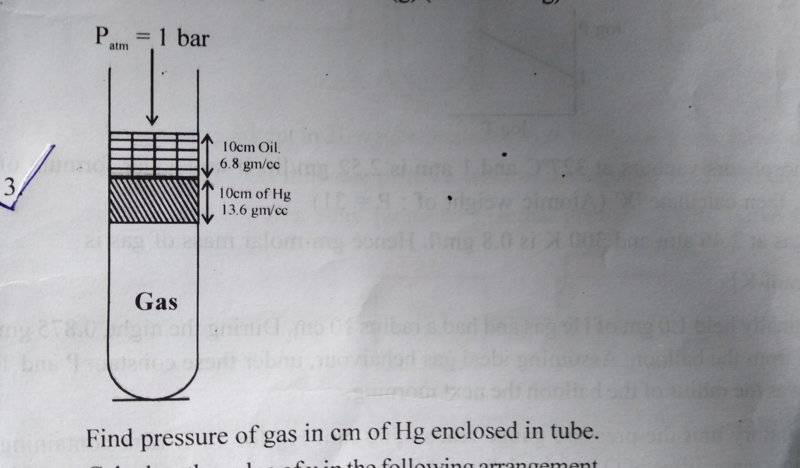# Is P(atm)=P(gas)?

• navneet9431

Gold Member

## Homework StatementSee this question.

## Homework Equations

Pressure=Height*Density*Gravity

## The Attempt at a Solution

I think P_atm=P_gas.
I think so because for the two liquids(oil and Hg) to be in equilibrium the net force on them should be zero.
And,since they are not getting displaced anywhere from their position.So,they are in equilibrium.Hence,the force exerted by atmosphere from upward should be equal to the force exerted by the Gas from downward.
But,if I solve the question using this concept,I get wrong answer every time.
Can you please tell me where is my this logic wrong?
I will be thankful for help!
Note:English is my second language.

#### Attachments

Last edited:

1 atm=76.0 cm of Hg. (Usually known as 760 mm of Hg). In addition you have 10 additional cm of Hg, along with 10 cm of oil that has 1/2 the density of Hg, so it is the equivalent of 5 cm of Hg. You should be able to compute ## P ## now in terms of centimeters of Hg, and then convert it to atmospheres. Although you could work this problem by calculating gravitational forces, that conversion is unnecessary. The upward pressure must equal the sum of the downward air pressure plus additional downward forces per unit area.

Can you please tell where was my logic wrong?
1 atm=76.0 cm of Hg. (Usually known as 760 mm of Hg). In addition you have 10 additional cm of Hg, along with 10 cm of oil that has 1/2 the density of Hg, so it is the equivalent of 5 cm of Hg. You should be able to compute ## P ## now in terms of centimeters of Hg, and then convert it to atmospheres. Although you could work this problem by calculating gravitational forces, that conversion is unnecessary.

See also the sentence or two that I added above. Gravity exerts a downward force on the liquids, which is proportional to their masses. You can think of the mass as simply being weight, just like pressure in centimeters of Hg is really using mass for the unit of force rather than converting it to the force in dynes by multiplying it by ## g=980 ##cm/sec^2. (and you don't need to use the fact that the density of Hg is 13.6 g/cm^3). They are actually simplifying the problem for you, perhaps too much so, and thereby causing some confusion.

Sorry,But I am not getting it.
Since the two liquids(Hg and Oil) are at equilibrium.
So,the Force_Atmosphere=Force_Gas!
Hence P_atm=P_gas!
Do you think it is wrong?
Note:English is my second language.
See also the sentence or two that I added above. Gravity exerts a downward force on the liquids, which is proportional to their masses. You can think of the mass as simply being weight, just like pressure in centimeters of Hg is really using mass for the unit of force rather than converting it to the force in dynes by multiplying it by ## g=980 ##cm/sec^2. (and you don't need to use the fact that the density of Hg is 13.6 g/cm^3). They are actually simplifying the problem for you, perhaps too much so, and thereby causing some confusion.

If you have a test tube/cylinder of cross-sectional area of 1 cm^2, and you put Hg in it that goes to a height of 10 cm, how much does the Hg weigh in grams? Why is it that it requires a force to lift that test tube? It even requires a force to hold it up so that it doesn't fall to the ground. ## \\ ## The Hg has a downward force on it from gravity. This force must be balanced or the Hg would accelerate downward by F=ma=mg. In your above problem, the pressure ## P ## supplies this upward force, to balance the gravitational force, along with the downward force from the air pressure above.

If you have a test tube/cylinder of cross-sectional area of 1 cm^2, and you put Hg in it that goes to a height of 10 cm, how much does the Hg weigh in grams? Why is it that it requires a force to lift that test tube? It even requires a force to hold it up so that it doesn't fall to the ground. ## \\ ## The Hg has a downward force on it from gravity. This force must be balanced or the Hg would accelerate downward by F=ma=mg. In your above problem, the pressure ## P ## supplies this upward force, to balance the gravitational force, along with the downward force from the air pressure above.
And this pressure is being supplied by the gas.Right?

•If an elephant is sitting on you (beneath the atmosphere above), and the elephant is in equilibrium, then the only force acting on you is from the atmospheric pressure?

## Homework Statement

View attachment 229751
See this question.

## Homework Equations

Pressure=Height*Density*Gravity

## The Attempt at a Solution

I think P_atm=P_gas.
I think so because for the two liquids(oil and Hg) to be in equilibrium the net force on them should be zero.
And,since they are not getting displaced anywhere from their position.So,they are in equilibrium.Hence,the force exerted by atmosphere from upward should be equal to the force exerted by the Gas from downward.
But,if I solve the question using this concept,I get wrong answer every time.
Can you please tell me where is my this logic wrong?
I will be thankful for help!
Note:English is my second language.
Have you drawn any free body diagrams of the mercury and the plug, or do you think you have advanced beyond the point where you no longer need to use free body diagrams?